## The weights of newborn baby boys born at a local hospital are believed to have a normal distribution with a mean weight of 3903 grams and a

Question

The weights of newborn baby boys born at a local hospital are believed to have a normal distribution with a mean weight of 3903 grams and a standard deviation of 446 grams. If a newborn baby boy born at the local hospital is randomly selected, find the probability that the weight will be less than 4884 grams. Round your answer to four decimal places.

in progress 0
3 months 2021-08-07T20:29:41+00:00 1 Answers 22 views 0

0.9861 = 98.61% probability that the weight will be less than 4884 grams.

Step-by-step explanation:

Normal Probability Distribution:

Problems of normal distributions can be solved using the z-score formula.

In a set with mean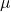and standard deviation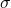, the z-score of a measure X is given by: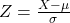The Z-score measures how many standard deviations the measure is from the mean. After finding the Z-score, we look at the z-score table and find the p-value associated with this z-score. This p-value is the probability that the value of the measure is smaller than X, that is, the percentile of X. Subtracting 1 by the p-value, we get the probability that the value of the measure is greater than X.

Mean weight of 3903 grams and a standard deviation of 446 grams.

This means that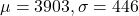If a newborn baby boy born at the local hospital is randomly selected, find the probability that the weight will be less than 4884 grams.

P-value of z when X = 4884. So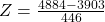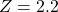has a p-value of 0.9861

0.9861 = 98.61% probability that the weight will be less than 4884 grams.